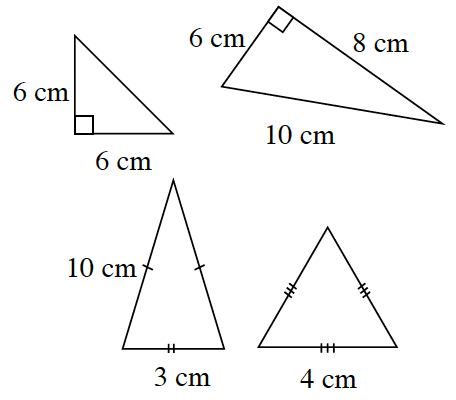### Home > INT2 > Chapter 5 > Lesson 5.2.3 > Problem5-94

5-94.The four triangles at right are placed in a bag. If you reach into the bag without looking and pull out one triangle at random, what is the probability that:

1. The triangle is scalene?

Remember that a scalene triangle has no sides of equal length.

2. The triangle is isosceles?

Remember that an isosceles triangle has two sides of equal length.

3. At least one side of the triangle is $6$ cm?

For part (c) use the formula: $\frac{\text{number of triangles that have at least one side of 6 cm}}{\text{total number of triangles}}$

$\text{P}\text{(side of triangle is 6 cm)}=\frac{2}{4}=\frac{1}{2}$